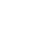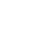Home » Teaching Multiplication Virtually? Try these helpful and easy ideas!

# Teaching Multiplication Virtually? Try these helpful and easy ideas!

If you’re teaching multiplication virtually let me show you how with physical manipulatives, a document camera, and virtual manipulatives.

Teaching the concept of multiplication has always been a highlight of the year while teaching third grade. The move from additive reasoning to a new kind of mathematical reasoning (multiplicative reasoning) is exciting to watch grow. Teaching multiplication virtually puts a lot of pressure on the teacher to successfully develop this concept in her students!

Estimated reading time: 19 minutes

But I hope that with this post, I can show you how to develop the concept of multiplication, develop mathematical reasoning in your students while using the same successful techniques of in-person teaching applied to a virtual setting. The most important point to remember is to make the math visual!

## Teaching Multiplication Virtually – First Things First

To teach multiplication virtually, we must first understand what multiplication is. Most teachers will quickly connect repeated addition to multiplication facts (3 x 4 = 3 + 3 + 3 + 3). Even second graders learn to “use addition to find the total number of objects arranged in a rectangular array.” (CCSS, 2.OA.C.4). In reality, you are showing a different way to write the expression 3 x 4 by using a different operation. Multiplication is not repeated addition.

What! The idea of multiplication as repeated addition does not hold up with multiplying one fraction to another, so we should not mislead our students.

Instead, think of multiplication as scaling. You can scale up (multiplying integers) or scale down (multiplying fractions). And this is the essence of multiplicative reasoning – understanding proportional situations. We’re going to use this notion of scaling and proportion to teach multiplication virtually!

## Teaching Multiplication Virtually – Which Methods?

I’ve described the three best hands-on and visual ways to teach multiplication in a previous post. Those same three methods can and should be done virtually – equal groups, arrays, and number lines. These ways of visually modeling multiplication align closely with the idea of scaling numbers. Remember, make the math visual!

To start teaching multiplication virtually, start math instruction with a context – preferably a real-world, relatable context for your students.

Here’s a relatable situation for elementary students.

My favorite video game to play is Fortnite. But my parents will only let me play 12 hours max for the week. If I play more than that, they’ll take it away! So, I played Fortnite 3 hours a day for 4 days. Did I reach the max? Did I go over? Do I have any time left?

If teaching in person, I would normally have students use beans and cups to visually see what is happening in the problem. To teach multiplication virtually, I would now use Zoom (or Google Meets) and a document camera. My district has a district license for Zoom as well as issuing me an Ipevo document camera. I use the Ipevo software called Visualizer. It’s available for free for Mac, PC, iOS, and Android. Use any other free document camera Apps and software available.

## What Materials will the Students Need?

Before the lesson, I would ask students to gather objects that can be used as manipulatives. These could be beans, buttons, pennies, small macaroni, etc. They need to be uniform in size and shape. Anywhere from 50 to 100 of these should be fine. I would also suggest they gather at least 10 small styrofoam cups, plastic cups, or Dixie type cups.

For those students who cannot gather the materials, I’ve put together a Make Multiplication Visual printable PDF that includes cups and squares that students can use in place of the real thing. They’ll need to be printed on cardstock or regular paper and cut out. Sign up for my newsletter and get the Make Multiplication Visual printable PDF for FREE!

If the student can not gather the physical objects or print and cut out the PDF’s printables, then your best bet is for the student to use a blank paper to draw circles and dots (or stars). My preference is for using real objects. Physically counting, moving, or sorting objects helps with making sense of the math, whether in person or teaching multiplication virtually.

## Making Equal Groups

Please start with the context like the one previously shared while having students discuss what they think about the situation.

• Is it a fair arrangement?
• Will the number of allowed hours be exceeded? How do you know?
• How can I visually show how much time I spent playing video games for one day using beans and cups?

Using the Visualizer App on my laptop in concert with the document camera, I can share my screen on Zoom. Remember, make math visual!

• On the document camera, put one cup to show one day of playing video games. By the way, I use a felt background, so beans or other objects don’t roll away!
• Then in the cup, put 3 beans counting each as an hour played that day.
• Now ask: If I did the same thing on the second day, how would I show that?
• Most students will say, you put another cup and 3 more beans.
• Add the second, third, and fourth cups in the same way as before

The key question to ask is how do the 2 (or 3 or 4) cups compare? You hope to hear language such as you’ve doubled it; you have twice as much; there are 2 times as many, etc. This type of vocabulary describes multiplicative thinking. If your students don’t suggest these terms, then definitely bring them up and discuss why they are appropriate.

You might also have a student say you ADDED another 3 beans which is mathematically correct. And if I added another three beans, etc., I could say I’m increasing each sum by three. But this is additive reasoning.

We want to move the students to multiplicative reasoning. So when a student says you added another group, you could then redirect with: another way to say it is I doubled the amount or tripled the amount, or I now have 4 times the amount. We use these terms because each group has the same quantity or value.

Using terms such as doubling, tripling, 4 or 5 or 6 times as much, you are now scaling numbers, not adding them. Later, when students start to multiply fractions, they will see scaling go in the opposite direction because the product will be smaller in value than the factors.

## Using the Same 12 Beans – BUT with a New Context

In the previous example of teaching multiplication virtually, I used the factors 3 and 4 to make 12. But are those the only whole factors which make 12? Nope. You can also multiply 6 and 2 or 12 and 1.

The next step is to ask students to figure out another way to play the 12 hours of game time, with the criteria you have to play the same number of hours daily. What’s another way to reach 12 hours of total video game time if I played the same amount of hours every day?

Let students use their cups and beans to figure out how they can equally distribute those 12 hours. Remember the Commutative Property of Multiplication will apply as you will have one student show 2 days with 6 hours each and another 6 days with 2 hours each. More on this property when we introduce arrays!

Once students understand how to distribute a set number of beans equally, you can start giving them some practice in which they have to represent the following situations with the beans and cups. Offer several practice problems for each situation using a variety of factors.

• How much is 2 groups of 5?
• I have 3 beans. What is 6 times as many?
• I have 5 beans. What is twice (or triple) the amount?

In another lesson, you can introduce the academic vocabulary of factors, product, multiple, etc. When you feel certain students are grasping the concept of multiplication using equal groups, start introducing the expressions to match the situations.

## Moving on to Arrays to Teach Multiplication Virtually

When I was in the physical classroom, I took out 24 tiles (we had foam tiles as part of our math manipulative kits). I asked my students what is a way to make the counting more efficient to find the total? They suggested grouping by a certain quantity to make groups that could be skipped counted. But I pointed out it’s hard to know if all my groups are equal by looking at them. One student suggested putting the tiles in a row. Bingo! Students started making rows to begin constructing arrays.

When they finished, I asked, how can you know each group is equal? The response usually is each ROW or COLUMN is equal because the tiles matched up to other rows or columns. I explained mathematicians find ways to do math more efficiently – meaning with fewer steps. So now I formally introduce the concept of arrays. I make sure the students understand the difference between rows and columns.

I have them practice making arrays using tiles, paper squares, or even beans (or whatever manipulative they have). Remember, make the math visual! We examine how arrays look, matching rows and columns to factors. Someone eventually discovers or declares 3 x 4 is the same as 4 x 3. Another Bingo!

By the way, showing everyday objects in an array makes an effective number talk! How did you see the ______? This will reinforce the concept of rows and columns. You can do a Google Image search for pictures of everyday objects arranged in an array. Put the images in a Google Slides™ to screen share in Zoom. Show the image for 3 – 5 seconds. Then have students say how many they saw and how did they see the objects.

## The Commutative Property of Multiplication

Even if you are teaching multiplication virtually, this important property of multiplication needs to be taught. If you are using tiles or paper squares, use it to teach multiplication as an area model. You can also use graph paper. On graph paper, students can make a 3 x 4 array and a 4 x 3 array. Then cut each out and stack one atop the other to prove the Commutative Property does work!

I’ve also included possible pre-made arrays in the Make Multiplication Visual printable PDF. You can make your own arrays using the table function in PowerPoint or Google Slides.™ Cut them out and display them under the document camera. Rotate an array to show how the factors may change order, but the product or area remains the same.

You can share a real-life context by thinking about the placement of a bed in a bedroom. A bed is usually a rectangular shape. You can move this rectangular shape or rotate it, but your bed remains the same size.

In my Teachers Pay Teachers store, I also have many resources which I have used every year to teach all the Properties of Multiplication. Many are easily adaptable to virtual teaching situations such as animated PowerPoints and posters.

## Multiplication and Number Lines – a Length Model

I remember the first time I had tried teaching multiplication with number lines. It was a hot mess. Why? At the time, I didn’t understand completely how it related to multiplication. Arrays represent an area model of multiplication (as well as fractions). But a number line represents a distance or interval from one number to another.

One way to transition to number lines is to use tiles or paper squares. For example, with 3 x 4, I can lay out 3 tiles in a row. The factor of 4 means I have to create another row which is 4 times as long. It’s reminiscent of a bar model, but what you’re visually seeing is a length model for multiplication. Remember, make math visual!

If I walk 3 miles a day, how many will I walk in 4 days? That’s 4 times the distance. When using number lines, it’s more effective to use contexts which involve measurement. I used 3 cups of flour to make a dozen cookies. If I make 4 dozen cookies, how much flour will I need?

The number line you use can be open or marked with intervals. Start with the marked interval number lines since this will help students see the scale of the jump or distance between intervals.

Start with a context such as the ones above.

• Use a colored pencil or marker to mark the distance from zero to the first factor (let’s say we use 3 x 4).
• Mark a dot on the 3 on the number line.
• Then color in the distance from zero to 3.
• Then use a different color to show how much 4 times as many would be. If I walked 3 miles in one day, how can I show 4 days or 4 times as much?
• Start at zero and mark 3 miles each time on 3, 6, 9, and 12.
• Then draw a line from zero to 12. Now you can visually see how 3 x 4 is 4 times the length of 3.

The intervals of 0 -3, 3 – 6, 6 – 9, 9 – 12 are usually referred to as jumps. They’re usually drawn as an arc from one number to the next. I have used this method, as well. However, I have found it to be sometimes confusing for some kids. Starting with a length model by drawing a straight color line seems to be less confusing. But you can use both methods.

Eventually, you’ll want to move your students to an open number line. Open number lines help students understand equal jumps or scaling since they will determine the length between each jump – and they should be equal jumps or lengths!

## Using Virtual Manipulatives to Teach Multiplication Virtually

Using virtual manipulatives to teach multiplication can be a little tricky. With too many movable pieces or having to add individual pieces, instructional time can be compromised. Building equal groups, arrays, or working on a number line can require additional time. Below I have listed some of the best virtual manipulatives for teaching multiplication virtually (without losing instructional time!).

You can use the same methods (equal groups, arrays, and number lines) with the following virtual manipulative sites. Please note some require Flash to run. According to Adobe®, Flash will be discontinued on December 31, 2020. It will still run, but there will be no additional updates. So some sites requiring Flash may no longer work. Test each site before using it to make sure it is compatible with your device.

### iTools by Houghton Miflin

This virtual manipulative site runs on Flash but has some of the best math tools to show multiplication visually. Using their virtual counters, the tool can form equal groups. It visually shows the equal groups as well as the standard notation.

You can also use the counting tool to make arrays. However, there is not an annotation tool unless you use the annotation tools in Zoom.

One of the best tools available on iTools is the number line. Using it for multiplication, the tool animates the jumps on the number line!

### mathlearningcenter.org

This virtual manipulative site has a spate of handy virtual tools. Their Number Line App (web-based or iOS and Android) works well to show multiplication as a length model. You can use the built-in annotation tools to draw the lengths as well as write the expression.

The Base-Ten Blocks App can be used to create arrays (make one row, select each cube, then use the duplicate function at the bottom in the tool ribbon). You can use the annotation tool to write expressions or even prompts.

One of the most powerful features of many of the Apps on The Math Learning Center site is the ability to share whatever you create with your students. Create an array, write a prompt, then share it with your students.

### mathsbot.com

This site has a large selection of math tools. One particular tool is Multiplication Areas, which allows you to visually build an area model (arrays). No annotation tool is available, but it can be used to show the Commutative Property.

Another Mathsbot tool is the Scaled Times Tables. This tool visually shows the area in a configuration similar to a multiplication chart. Because the values are scaled, it is a powerful tool to prompt discussions about multiplication.

### PhET Simulations

PhEt Simulations is well known for science-based simulations. But did you also know they have many math simulations as well? One in particular is effective for showing the area model. Build arrays in any configuration with any multiplication expression.

Unfortunately, there are no annotation tools built into this tool.

### NCTM Interactives

The National Council of Teachers of Mathematics is a premier resource for math educators. Available for free is the NCTM Interactives. One particular interactive is Bunny Times Interactive. It uses visual models to build a conceptual understanding of multiplication. Use it as a teaching tool or assign it as a game.

## Teaching Multiplication Virtually – Final Thoughts

If you teach equal groups, arrays, and number lines to your students, will they see that these representations are connected? It’s important to connect these visual representations one to the other so they understand multiplication can be represented in many ways. Remember, make the math visual!

One way to do it is to provide students with a problem to solve. Have them share how they solved it through drawings, Google Slides, screenshots, etc. Then put together a slideshow with different ways students solved the same problem. Then ask students to compare the different ways students solved the problem. What’s the same? What’s different?

## Teaching Multiplication Virtually – Some More Resources

Over the years, I have created resources for teaching multiplication – from concept to practice. Below I’ve highlighted some resources which lend themselves well to distance learning situations.

Teaching the Concept of Multiplication PowerPoint is a fully animated and interactive PowerPoint which can be screen shared with students over Zoom or Google Meet. It shows a visual representation of multiplication through equal groups, arrays, and number lines.

Properties of Multiplication PowerPoint is a fully animated and interactive PowerPoint which can be screen shared with students over Zoom or Google Meet. It covers the Zero Property, Identity Property, Commutative Property, and Associative Property of Multiplication.

Distributive Property of Multiplication PowerPoint is a fully animated and interactive PowerPoint which can be screen shared with students over Zoom or Google Meet. Students will learn how to split arrays, write a distributive property sentence, and solve.

Distributive Property of Multiplication Practice on Google Slides™ is an interactive digital notebook with 26 slides which includes practice problems at a depth of knowledge levels 1 to 3.

Multiplication Practice Pumpkin Patch Game is a self-paced and self-checking Google Slides™ game which helps students practice with equal groups, arrays, and number lines. The game includes 25 problems.Multiplication Practice on Google Slides
Multiplication Practice on Google Slides
Multiplication Practice on Google Slides
Multiplication Practice on Google Slides
Multiplication Practice on Google Slides
Multiplication Practice on Google Slides
Multiplication Practice on Google Slides
Multiplication Practice on Google Slides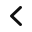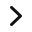## Sign up to Get the FREE Make Multiplication Visual printable PDF!

When you sign up for my newsletter I’ll send you a link to download a PDF containing the following:

• cups
• 100 grid of squares
• arrays for 12, 16, 18, 20 and 24
• multiplication chart
• number lines

Since it’s a PDF, you can send it to students through email or post it in Google Classroom (or other LMS). Students can print the pages at home. You can also print it out and copy it to put together packets for students to pick up.

Remember, make the math visual!

What are your ideas for teaching multiplication virtually?

Share them in the comments!###### Follow:
Two Boys and a Dad

Hi, I’m Claudio, the dad from Two Boys and a Dad. Whether you’re just starting out teaching or a veteran teacher, let me offer you some ideas, tips, suggestions, resources and a sounding board for your daily classroom struggles (and successes!). This is your place to find information and ask questions about teaching in the elementary grades.

Find me on: Web | Twitter | Instagram | Facebook

Share:

### Interactive Digital Notebooks for Google Slides®Digital Notebooks for GeometryLIfecycle Digital Notebooks for Google SlidesDigital Notebooks for Math, Science and Social Studies
Digital Notebooks for Geometry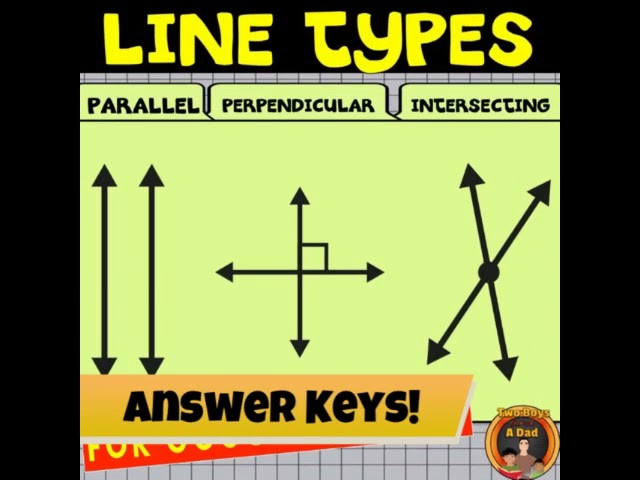LIfecycle Digital Notebooks for Google SlidesDigital Notebooks for Math, Science and Social Studies